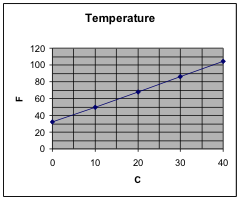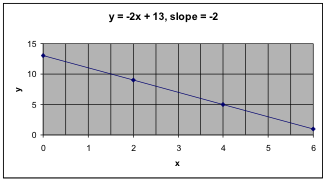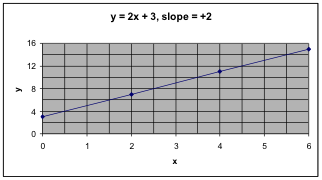## G1.01: Intro & Slope

### Learning Objectives

1. Understand slope of a line in two ways (graphically by rise/run, algebraic formula in x’s and y’s.)
2. Understand the y-intercept of a line in two ways (graphically as the intercept of the y-axis, algebraic formula in x’s and y’s.)
3. Algebraically, find the slope when given two points.
4. Write the formula for the line in standard slope-intercept form and notice which number is the slope and which number is the y-intercept. Interpret the slope and intercept.
5. Find the formula for a line when given the slope and one point on the line.
6. Find the formula for a line when given two points on the line.
7. Find the inverse formula, when needed, by interchanging the input and output variables. Notice the relationship between the slopes of the formula and the inverse formula.
8. Use a spreadsheet to explore the effects on the graph of changing the values of the slope and intercept.
Summary of algebraic facts and interpretations:

Slope:

The slope of a line is $m=\frac{rise}{run}=\frac{{{y}_{2}}-{{y}_{1}}}{{{x}_{2}}-{{x}_{1}}}$, where the two points $({{x}_{1}},{{y}_{1}})$ and $({{x}_{2}},{{y}_{2}})$are on the line.

Slope-intercept form:

If the formula is $y=mx+b$

where y and x are the variables and m and b are numbers, then the graph of this formula is a straight line with slope m and y-intercept b.

This can also be written as $y=b+mx$

Point-slope form:

If the slope of a line is m and one point on the line is $({{x}_{0}},{{y}_{0}})$, then the equation of the line can be written as $y-{{y}_{0}}=m(x-{{x}_{0}})$ which gives the formula:

$y=m(x-{{x}_{0}})+{{y}_{0}}$

Interpret the slope:

m is the amount we expect y to increase when x increases by 1.

Interpret the intercept:

b is the value of y when the value of x is zero.

We want to investigate the relationship between temperature Centigrade and temperature Fahrenheit in order to take temperatures from a news broadcast in Canada (in Centigrade) and convert them to temperatures that are easy for US citizens to understand (in Fahrenheit.) We already know the formula for that and we know how to find points, plot them, and draw a graph.   (See Topic C: Formulas.)

Here, the temperature Centigrade is the input value and the temperature Fahrenheit is the output value. The formula is $F=1.8\cdot{C}+32$ and following is a table of values and a graph.C F 0 32 10 50 20 68 30 86 40 104

## Slope

Notice that the graph appears to be a straight line. That suggests that this is a linear relationship. A relationship is linear if the same increase in the input always leads to the same increase in the output.   We can look at the table of values and see that, for every increase in C of 10°, there is an increase of 18° in F.

In general, for a straight line with input variable x and output variable y, the slope is defined to be   slope = $m=\frac{rise}{run}=\frac{{{y}_{2}}-{{y}_{1}}}{{{x}_{2}}-{{x}_{1}}}$, where the two points $({{x}_{1}},{{y}_{1}})$ and $({{x}_{2}},{{y}_{2}})$are on the line.

In the temperature example, we notice that the variable C plays the role of x and the variable F plays the role of y. So we can compute the slope of the line using the two points (0,32) and (10,50). Call the first point $({{x}_{1}},{{y}_{1}})$ and the second point $({{x}_{2}},{{y}_{2}})$.

Slope = $m=\frac{rise}{run}=\frac{{{y}_{2}}-{{y}_{1}}}{{{x}_{2}}-{{x}_{1}}}=\frac{50-32}{10-0}=\frac{18\,\,\,degrees\,\,F}{10\,\,\,degrees\,\,C}=1.8$ degrees F per degree C.

For this to be a linear relationship, the slope must be the same no matter which two points on the graph you use to compute it. Let’s try one more pair of points.   So we can compute the slope of the line using the two points (20,68) and (40,104). Call the first point $({{x}_{1}},{{y}_{1}})$ and the second point $({{x}_{2}},{{y}_{2}})$.

Slope = $m=\frac{rise}{run}=\frac{{{y}_{2}}-{{y}_{1}}}{{{x}_{2}}-{{x}_{1}}}=\frac{104-68}{40-20}=\frac{36\,\,\,degrees\,\,F}{20\,\,\,degrees\,\,C}=1.8$ degrees F per degree C.

There are several more pairs of points from our table of values that you could easily try. Try some of those and see if you get the same slope each time.

### Negative slope and positive slope.

Recall from algebra class that lines which are slanted down have negative slope and those slanted up have positive slope. One important method of checking your work in computing the slope is to be sure that your graphs and algebra give results that are consistent with that.

 y = 2x + 13y = 2x + 3